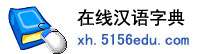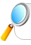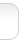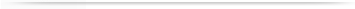汉字   按部首检索  按拼音检索王盟 张盟 李盟 陈盟 周盟 刘盟 杨盟 吕盟 孙盟 徐盟 吴盟 马盟 郭盟 崔盟 高盟 易盟 邵盟 贾盟 魏盟 谭盟 范盟 隋盟 连盟 佟盟 倪盟 余盟 陶盟 蔡盟 孟盟 于盟

 盟字的男姓名 | 盟字的女姓名 | 盟开头的姓名 | 盟结尾的姓名 | 更多带盟的姓名
 盟 (男) 盟 (女) 联盟 (男) 盟盟 (女) 建盟 (男) 晓盟 (女) 盟盟 (男) 连盟 (男) 新盟 (男) 盟尧 (男) 盟君 (女) 海盟 (男) 晓盟 (男) 庆盟 (男) 世盟 (男) 盟飞 (男) 同盟 (男) 盟刚 (男) 宏盟 (男) 泽盟 (女) 秋盟 (男) 盟伟 (男) 国盟 (男) 小盟 (女) 盟岚 (女) 章盟 (男) 盟宇 (男) 盟然 (男) 瑞盟 (男) 智盟 (男) 永盟 (男) 盟钦 (男) 以盟 (男) 金盟 (女) 友盟 (女) 盟姝 (女) 雅盟 (女) 盟军 (男) 岁盟 (男) 盟慈 (女) 恩盟 (男) 盟萌 (女) 盟明 (男) 盟懿 (男) 佳盟 (男) 温盟 (男) 思盟 (女) 洪盟 (男) 盟雅 (女) 泽盟 (男) 盟晓 (男) 盟杰 (男) 盟天 (女) 盟东 (男) 士盟 (男) 天盟 (男) 钦盟 (男) 利盟 (男) 宇盟 (男) 盟雯 (女) 伟盟 (男) 一盟 (男) 萌盟 (女) 友盟 (男) 先盟 (男) 盟钰 (女) 盟础 (男) 展盟 (男) 凤盟 (男) 盟舜 (男) 绍盟 (男) 盟安 (男) 胜盟 (女) 子盟 (男) 育盟 (女) 林盟 (男) 颖盟 (男) 盟夭 (男) 廷盟 (男) 祝盟 (男) 献盟 (男) 琮盟 (男) 盟媛 (女) 正盟 (男) 秋盟 (女) 东盟 (男) 昌盟 (男) 盟晴 (女) 联盟 (女) 亚盟 (男) 盟海 (男) 盟羿 (男) 盟煌 (男) 盟婷 (女) 盟凯 (男) 盟仁 (男) 紫盟 (男) 盟盯 (女) 兆盟 (男) 盟速 (女) 盟欢 (男) 辰盟 (男) 盟川 (男) 伯盟 (男) 盟臣 (男) 思盟 (男) 若盟 (男) 凡盟 (男) 盟初 (男) 道盟 (男) 彰盟 (男) 可盟 (男) 寿盟 (男) 相盟 (男) 少盟 (男) 盟贵 (男) 小盟 (男) 盟天 (男) 杰盟 (男) 殿盟 (男)

 版权所有　在线汉语字典   浙ICP备05019169号